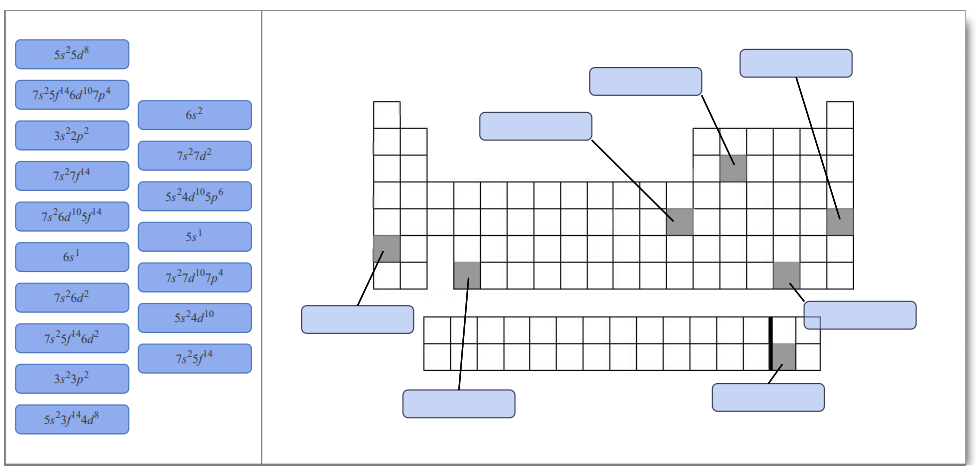# Problem: The black line between elements 56 and 72 in the periodic table shown indicates that in the Lanthanide series elements 57 through 71 are listed below the main table, while in the Actinide series elements 89-103 are listed below the main table. Elements 72 and 104 are listed in main table. Identify the outer electron configuration of each element shown in this periodic table outline.The periodic table lists all known elements arranged by atomic number. Atomic number is the nuclear charge, the number of protons in the nucleus of an an atom of a particular element. For a neutral atom, the number of protons is equal to the number of electrons. Each column of the table, called a group, contains elements with the same number of valence electrons that are in different quantum levels. Each row of the table, called a period, contains elements with differing numbers of valence electrons that are in the same principal quantum level. The four main blocks of the table (s, p, d, and f) contain elements whose highest energy electrons have the same azimuthal quantum number (ℓ).

###### FREE Expert Solution
79% (161 ratings)View Complete Written Solution
###### Problem Details

The black line between elements 56 and 72 in the periodic table shown indicates that in the Lanthanide series elements 57 through 71 are listed below the main table, while in the Actinide series elements 89-103 are listed below the main table. Elements 72 and 104 are listed in main table.

Identify the outer electron configuration of each element shown in this periodic table outline.The periodic table lists all known elements arranged by atomic number. Atomic number is the nuclear charge, the number of protons in the nucleus of an an atom of a particular element. For a neutral atom, the number of protons is equal to the number of electrons. Each column of the table, called a group, contains elements with the same number of valence electrons that are in different quantum levels. Each row of the table, called a period, contains elements with differing numbers of valence electrons that are in the same principal quantum level.

The four main blocks of the table (s, p, d, and f) contain elements whose highest energy electrons have the same azimuthal quantum number (ℓ).